﻿ 固体氧化物燃料电池–燃气轮机混合动力系统结构分析及性能综合评价 System Structure Analysis and Fuzzy Comprehensive of Fuel Cell (SOFC)-Gas Turbine Top Circulation

Dynamical Systems and Control
Vol.07 No.03(2018), Article ID:26111,6 pages
10.12677/DSC.2018.73026

System Structure Analysis and Fuzzy Comprehensive of Fuel Cell (SOFC)-Gas Turbine Top Circulation

BaiHui Xu1,2, Jimin Ren2, Qianchao Liang2

1Naval 92089 Base, Ningbo Zhejiang

2College of Architecture and Power, Naval University of Engineering, Wuhan Hubei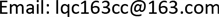Received: Jul. 1st, 2018; accepted: Jul. 20th, 2018; published: Jul. 27th, 2018ABSTRACT

This paper introduces four kinds of cyclic structures of the top-level model of fuel cell and gas turbine combined cycle power generation system, puts forward the method of applying fuzzy comprehensive evaluation to analyze the power generation system and judge the advantages and disadvantages of these four circulation modes, and then confirms the positive role of the intercooler, regenerator and supercharger.

Keywords:Top Circulation, Regenerator, Supercharger, Fuzzy Comprehensive Analysis

192089部队，浙江 宁波

2海军工程大学动力工程学院，湖北 武汉1. 前言

2. 燃料电池(SOFC)与燃气轮机混合系统的顶层循环结构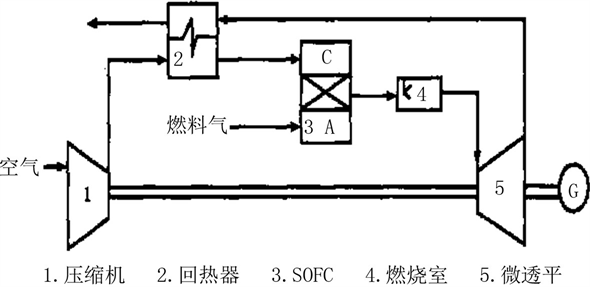Figure 1. The gas turbine of used regenerator and SOFC structure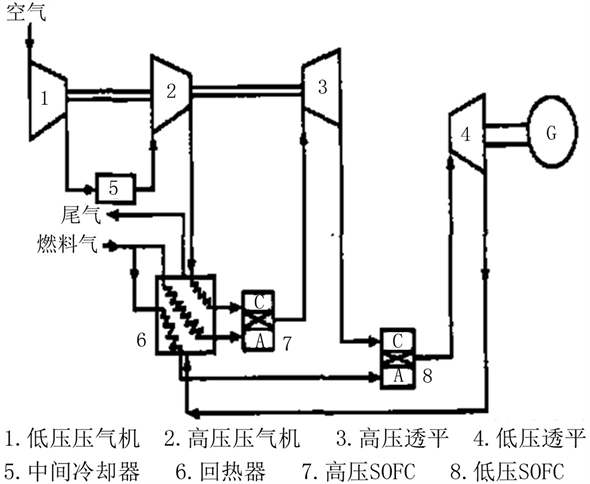Figure 2. The gas turbine of the intercooler-regenerator and SOFC structure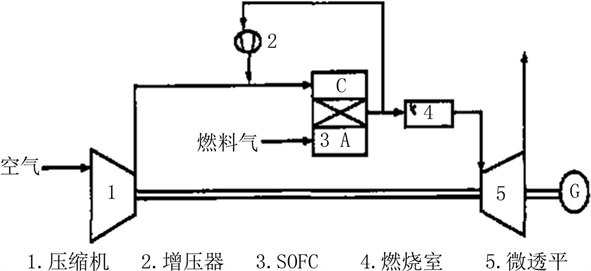Figure 3. The gas turbine of the supercharger and SOFC structureFigure 4. The gas turbine of the regenerator-supercharger and SOFC structure

3. 燃气轮机与燃料电池循环结构的模糊评判模型

$\sum _{i=1}^{m}{U}_{i}=U$$\left\{{U}_{i}\cap {U}_{j}=\Phi \left(i\ne j\right)\right\}$

${B}_{i}={A}_{i}\circ {R}_{i}=\left[{b}_{i1},{b}_{i2},\cdots ,{b}_{in}\right]$

$R=\left[\begin{array}{c}{B}_{1}\\ {B}_{2}\\ ⋮\\ {B}_{m}\end{array}\right]=\left[\begin{array}{cccc}{b}_{11}& {b}_{12}& \cdot \cdot \cdot & {b}_{1n}\\ {b}_{21}& {b}_{22}& \cdot \cdot \cdot & {b}_{2n}\\ ⋮& ⋮& \ddots & ⋮\\ {b}_{m1}& {b}_{m2}& \cdot \cdot \cdot & {b}_{mn}\end{array}\right]$

4. 系统循环结构模糊评判的应用实例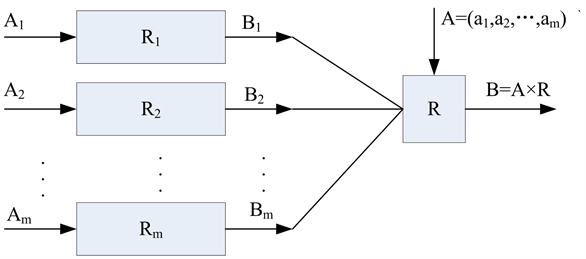Figure 5. The graphical symbol of two layer-fuzzy judgment

$\begin{array}{l}E=\left\{E1,E2,E3,\cdots ,E16\right\}\\ =\left\{0.090,0.090,0.090,0.066,0.066,0.066,0.066,0.066,\\ \text{\hspace{0.17em}}\text{\hspace{0.17em}}\text{\hspace{0.17em}}\text{\hspace{0.17em}}0.060,0.060,0.060,0.044,0.044,0.044,0.044,0.044\right\}\end{array}$

$u=\left\{u1,u2,u3,u4\right\}$ = {经济效益，占用体积，寿命，效率}

$A=\left\{A1,A2,A3,A4\right\}=\left\{0.23,0.15,0.11,0.51\right\}$

$V=\left\{V1,V2,V3,V4\right\}$

$\begin{array}{l}B1=A1\cdot R1=\left\{0.16,0.25,0.23,0.27\right\}\\ B2=A2\cdot R2=\left\{0.28,0.24,0.23,0.23\right\}\\ B3=A3\cdot R3=\left\{0.20,0.36,0.39,0.18\right\}\\ B4=A4\cdot R4=\left\{0.36,0.31,0.21,0.12\right\}\end{array}$

$Z=B×F=\left(0.484,0.249,0.161,0.106\right)×\left(90,75,60,50\right)=77.195$

5. 结论

System Structure Analysis and Fuzzy Comprehensive of Fuel Cell (SOFC)-Gas Turbine Top Circulation[J]. 动力系统与控制, 2018, 07(03): 239-244. https://doi.org/10.12677/DSC.2018.73026

1. 1. 冯登满, 刘畅, 杨苗苗, 等. 固体氧化物燃料电池的发展与研究[J]. 科技展望, 2016(5): 60.

2. 2. 耿孝儒, 吕小静, 翁一武. 基于生物质气的固体氧化物燃料电池–燃气轮机混合动力系统的性能分析[J]. 动力工程学报, 2015, 35(2): 166-172.

3. 3. 李杨, 翁一武. 固体氧化物燃料电池–燃气轮机混合动力系统及控制策略分析[J]. 中国电机工程学报, 2010, 30(35): 94-100.

4. 4. 黄刘松. 固体氧化物燃料电池–微型燃气轮机混合发电装置建模与仿真[D]: [硕士学位论文]. 上海: 上海交通大学, 2007.

5. 5. Tian, Y. (2002) Modeling of Solid Oxide Fuel Cell-Gas Turbine Hybrid Power Plant. Nanyang Technological University, Singapore.

6. 6. George, R.A. (2000) Status of Tub-ular SOFC Field Unit Demonstrations. Journal of Power Sources, 86, 134-139.

7. 7. 张化光, 邓玮, 耿加民. 发电用燃气轮机的非线性数学建模及稳定性分析[J]. 中国电机工程学报, 2007, 27(26): 108-114.

8. 8. 李政, 王德慧, 薛亚丽, 等. 微型燃气轮机的建模研究(I)-动态特性分析[J]. 中国动力工程学报, 2005, 25(1): 13-17.

9. 9. 李政, 王德慧, 薛亚丽, 等. 微型燃气轮机的建模研究——简化与分析[J]. 中国动力工程学报, 2005, 25(2): 160-164.

10. 10. 丁毅, 黄向华, 张天宏. 基于相似理论的燃气轮机建模技术研究[J]. 航空动力学报, 2004, 19(5): 589-694.